## Sunday, December 30, 2018

### Automatic Estimation of Signal Round Trip Delay, Part 2

In this post I explain how jack_delay estimator works. This utility is part of Linux JACK audio framework, its source code is available here.

The utility is designed to measure round trip delay of sound cards, it calculates the delay from the phase of the returned signal. The output and the input of the sound card need to be connected by means of a loopback cable: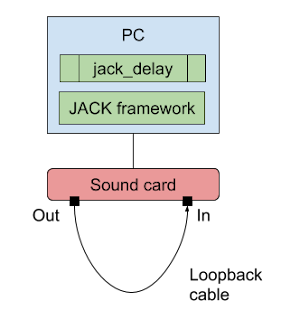This is how the estimator is hooked up in the program. Jack offers a processing callback which is called on a high priority (Linux "real time") dedicated thread, for low latency operation. This callback is registered by means of jack_set_process_callback API call. In the callback the delay estimator prepares and sends to output next audio buffer, and also receives a buffer of input data captured by the sound card. On the main thread the utility periodically checks and prints the current state of the delay estimator (mtdm):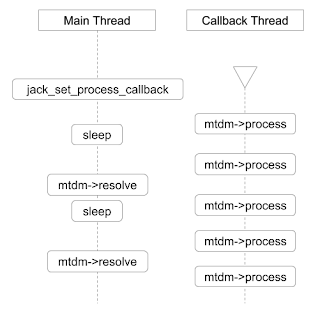What immediately occurred as strange to me is that the delay estimator class completely ignores the fact that it is being used in a multi-threaded setup—there are two threads that simultaneously read and write some of its fields, yet the latter are declared as ordinary variables. This gives the compiler a lot of freedom in rearranging the order of operations, which can affect correctness of the results. A correct modern solution would be to use atomic variables instead (but not a mutex, because the audio callback must call any function that could cause the thread to block, e.g. a function that attempts to acquire a mutex lock). Nevertheless, let's ignore this shortcoming of the implementation and proceed to the delay estimation algorithm.

The delay estimator uses 13 sine signals. The actual frequencies of those signals depend on the sampling rate. The algorithm uses an expression 2 * π * k * f / 65536 for calculating the phase in radians for each sample of the sine waves it generates. For the main sine wave f equals to 4096 which emits the expression 2 * π * k * 1 / 16—that is, a period of 16 samples. Thus, for the sampling rate of 48 kHz, the main sine has frequency of 48 / 16 = 3 kHz.

In order to measure the phase of the sine wave that has returned back, the algorithm uses Discrete Fourier Transform formula expressed via Euler's formula in terms of sine and cosine functions:

X(k) = Σ [n = 0..N - 1] x(n) * (cos(2π * k * n / N) - j * sin(2π * k * n / N))

The algorithm only needs the main harmonic of the signal, so it sets k to 1. The algorithm also swaps "cos" and "sin" terms, and thus uses sines instead of cosines as the eigenvectors. This is done for convenience, as using cosines would require shifting the calculated phase by π / 2 because the source signal is a sine wave. This is the corresponding part of the source code:

``````c =  cosf (a);
s = -sinf (a);
F->xa += s * vip;
F->ya += c * vip;``````

After obtaining real and imaginary parts of the frequency domain complex function value, the algorithm uses a simple low-pass filter two times in order to smooth out any abrupt changes in the signal's frequency domain form. Note—low pass filtering is not performed in the time domain, but rather in the frequency domain, thus the spectral composition of the signal remains the same.

The classical formula for a discrete time smoothing filter is:

y[i] = y[i - 1] + α * (x[i] - y[i - 1])

Below is the code where it is applied 2 times to real and imaginary parts:

``````F->x1 += _wlp * (F->xa - F->x1 + 1e-20);
F->y1 += _wlp * (F->ya - F->y1 + 1e-20);
F->x2 += _wlp * (F->x1 - F->x2 + 1e-20);
F->y2 += _wlp * (F->y1 - F->y2 + 1e-20);``````

The pair (x2, y2) is what ends up to be used to determine the phase of the sine wave. Note that the algorithm accumulates consecutive samples instead of building a smoothed vector. The smoothing parameter _wlp (same as α in the previous formula) is defined as 200 / sampling rate.

Since the algorithm doesn't try to determine the phase at a certain point, but rather pretends that the analyzed sine is of an infinite duration, its resolution goes as high as 16 / 65536 part of a sample (65536 steps for the phase distributed over 16 samples of the main sine wave's period). This is an extremely good resolution for all practical purposes!

But here we also have the multiplier ambiguity problem—since the sine function is periodic, the phase angle goes in circles, thus the maximum amount of phase shift that can be detected has the same time length as the period of the sine wave used. In other words, to detect a time delay of one second by using the phase of a single wave we would have to use a sine wave of 1 Hz frequency. Needless to say, it couldn't be well reproduced anywhere but in a purely digital system. And even then, we would have to use large DFT windows in order to capture an entire period of the wave. This is by all means impractical.

The program uses a really clever approach—each of the additional 12 sine waves helps to determine the value of the corresponding bit of the delay value. Thus the range of the delay measurement gets extended to (2 ** 12) * 16 = 4096 * 16 = 65536 samples, which is more than a second when 48 kHz sampling rate is used.

On the following graphs we can see how the phase rotation period doubles with each added sine wave. This is how the algorithm runs when it is using the first sine wave only: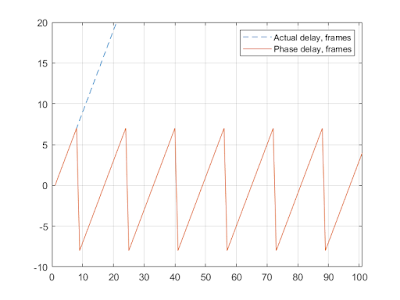As we can see, the phase of the sine wave follows the actual delay for the first 8 frames, then it resets to -8, grows back to 8, and so on. Now with the first two sine waves:The period has increased twice—to 32, and the values go from -8 to 24. Now with the first 3 sine waves:The period has increased twice once again, yielding the range of 64: from -8 to 56. And so on—when the algorithm uses all 13 sine waves the period becomes 65536 frames.

Let's now take a closer look at what happens inside the analysis loop. For the first sine wave the delay calculation is straightforward:

``d = atan2 (F->y2, F->x2) / (2 * M_PI);``

Phase, which is in the range from to π, is normalized into the range [-0.5, 0.5]. For every other sine wave, the following formula is used:

``p = atan2 (F->y2, F->x2) / (2 * M_PI) - d * F->f / f0;``

That's the phase of the current sine wave, minus the value of the delay calculated so far, scaled by the the ratio of the current frequency to the frequency of the main sine wave (f0). For the 4th sine wave, the value of p changes as shown on the following figure: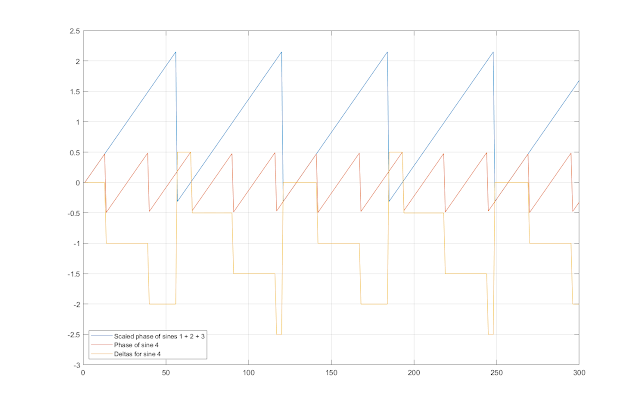Since the slopes of the phase functions match due to scaling, their difference is a step function. And because both phase functions are periodic, their difference is also periodic, with the period equal to the lowest common multiple of their periods. For example, the period of the combined wave formed by the sine waves 1, 2, and 3 is 64 samples. The period of the sine wave 4 (`_freq.f`) is 65536 / 2560 = 128 / 5 samples. Thus, the common period is 128 samples—that's 2 * 64 = 5 * 128 / 5—this can be easily seen on the figure—both two periods of the blue graph and five periods of the red graph comprise one period of the yellow graph.

Another interesting property of the resulting yellow graph is that the values of the first half of its steps are close to whole numbers: 0, -1, -2, and another half is close to halves: 0.5, -0.5, -1.5, -2.5. By using this fact, the algorithm transforms the step function into a square wave:Here is the corresponding code:

``````p -= floor (p);
p *= 2;
k = (int)(floor (p + 0.5));``````

First, the fractional part of the number is extracted. Note that because the values of the step function are not exactly whole numbers, and because they are negative, the resulting values may look surprising. For example, -0.0008179 gets "floored" to -1, and then, -0.0008179 - (-1) = 0.9992—as we can see, the red graph at X: 6 is almost 1. Anyways, the fractional part is a square wave with values very close to 0.5 or 1. The next two operations is doubling and rounding. The result is exactly 1 or 2—this is the blue graph. Note that depending on the input values, the fractional part can also have values close to 0 or 0.5, and the resulting square wave would be 0 or 1.

Below is the graph of the square wave functions calculated for the first four sine waves:By carefully choosing the frequencies of the sine waves, the resulting square waves always double their frequency at the next step. Another important property of the sine waves is that they always start with 0 or 2 value. This way, the algorithm simply determines whether the value of the square wave is divisible by 2, and uses the result to add the next power of 2 to the value of the calculated delay:

``````m = 1;
...
d += m * (k & 1);
m *= 2;``````

Thus, the main "magic" of the algorithm is the choice of the frequencies for the supplemental sine waves. In fact, we have already seen the required ratio:

2 * 2 ** (i + 3) = M * (65536 / _freq[i].f)

For example, for i = 3 (sine wave 4) we have:

2 * 2 ** 6 = 2 * 64 = 5 * (65536 / 2560) = 5 * (128 / 5)

M in this case is 5. The algorithm also works if M is 3 (thus having `_freq.f = 1536`). Below is a complete list of the ratios for all the sine waves used (the divisors of 65536 are the values from the the `_freq` array in the program code):

``````2 * 2 ** 4 = 2 * 16 = 1 * 32 = 1 * (65536 / 2048)
2 * 2 ** 5 = 2 * 32 = 3 * 64 / 3 = 3 * (65536 / 3072)
2 * 2 ** 6 = 2 * 64 = 5 * 128 / 5 = 5 * (65536 / 2560)
2 * 2 ** 7 = 2 * 128 = 9 * 256 / 9 = 9 * (65536 / 2304)
2 * 2 ** 8 = 2 * 256 = 17 * 512 / 17 = 17 * (65536 / 2176)
2 * 2 ** 9 = 2 * 512 = 17 * 1024 / 17 = 17 * (65536 / 1088)
2 * 2 ** 10 = 2 * 1024 = 41 * 2048 / 41 = 41 * (65536 / 1312)
2 * 2 ** 11 = 2 * 2048 = 97 * 4096 / 97 = 97 * (65536 / 1552)
2 * 2 ** 12 = 2 * 4096 = 225 * 8192 / 225 = 225 * (65536 / 1800)
2 * 2 ** 13 = 2 * 8192 = 833 * 16384 / 833 = 833 * (65536 / 3332)
2 * 2 ** 14 = 2 * 16384 = 1793 * 32768 / 1793 = 1793 * (65536 / 3586)
2 * 2 ** 15 = 2 * 32768 = 3841 * 65536 / 3841``````

The choice of the 'M' coefficient is more or less arbitrary. It needs to be non-divisible by 2, obviously. Also, the choice of M affects the frequency of the sine wave, thus M must be chosen so that the frequency stays in the required range, and is unique.

The remaining code in the app deals with possible phase reversal in the audio system and with noise. I will explore the robustness of the algorithm in the following post.

## Saturday, December 8, 2018

### Automatic Estimation of Signal Round Trip Delay, Part 1

When dealing with audio processing modules it might be important to know how much delay they introduce. This parameter is often called "latency." Typically we need to care about latency when using processing modules for real-time performance, or when they need to be synchronized with other audiovisual streams. Examples from my everyday applications are:

• my DSP computer running AcourateConvolver that I use as multichannel volume control with loudness normalization. Here I need to ensure that the delay introduced by AC does not exceed 100 ms to avoid noticeable lipsync issues while watching movies (yes, I could allow a longer delay and compensate for it on the video player side, but I use a lot of different sources: various players on the computer, BD player, XBox, and not all of them provide video delay);
• speaker synchronization in a multi-channel setup. In the simplest case, it's possible just to measure the distance from each speaker to the listening position, but if speakers use DSP (like LXmini), the processing delay must be taken into account, too.
• mobile devices and computers when used as real-time effect boxes for live instruments. In this case, the latency has to be quite low, ideally not exceeding 20 ms.

The module's delay is called "round trip" because the audio signal entering the processing module must eventually return back. With digital signal processing, the typical sources for delays are filters and various buffers that are used to reduce processing load and prevent glitching.

Measuring the round trip delay manually is a relatively easy task. The typical approach is to send a pulse through the processing box, capture it on the output, and somehow lay out the input pulse and the output pulse on the same timeline for measurement. This can be done either by using an oscilloscope, or with audio recording software, like Audacity. Below is an example of input and output impulses as seen on MOTU Microbook's bundled digital oscilloscope:Here, by eye we can estimate the delay to be about 25 ms. Needless to say, we need to use a pulse which doesn't get filtered out or distorted severely by the processing box. Also need to check the group delay of the box for uniformity, otherwise measuring latency at one particular frequency would not reveal the whole picture.

However, the manual approach is not always convenient, and I've spent some time researching automated solutions. From my experience with Android, I'm aware of several mobile applications: Dr. Rick'o'Rang Loopback app, AAudio loopback command-line app, and Superpowered Audio Latency Test app. On computers, there is a latency tester for the Jack framework—jack_delay. All these apps come with source code. What's interesting, they all use different approaches for performing measurements.

Yet another automatic delay measurement is bundled into ARTA and RoomEQ Wizard (REW), but their source code is not open. At least, for ARTA it's known that the delay estimation is based on cross-correlation between reference and measured channels.

I decided to compare different automatic approaches. The purpose is to figure out how reliable they are, and how robust they are when encountering typical kinds of distortions occurring in audio equipment: noise, DC offset, echoes, non-uniform group delay, signal truncation or distortion.

## Superpowered Audio Latency Test app

Let's start with the app that uses the most straightforward approach for round trip latency measurement. The source code and the description of the algorithm is located in this file. I'm referring to the state of the code tagged as "Version 1.7". The algorithm is designed to measure latency on the acoustical audio path of the device—from the speaker to the microphone. It can also be used with an electrical or a digital loopback, too.

At first, the algorithm must measure the average noise level of the environment. It does so over a 1 second interval (and for some reason, in the code the average of absolute sample values are called "energy", although in fact energy is defined in time domain as a sum of squares of sample values). The noise level is then translated into decibels, padded by 24 dB, and the resulting value is translated back into a 16-bit sample value, which is called the threshold.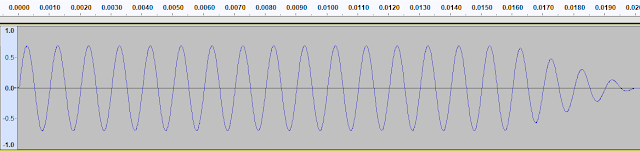Then the program outputs a pulse formed by a ramped down 1 kHz sine wave, 20 ms duration, with maximum loudness (the output level is set up manually via media volume on the device). On input, the algorithm waits for the first block of data where the average of absolute sample values exceeds the threshold, and within that block, finds the first sample exceeding the threshold. The index of this sample from the moment when the test pulse has been emitted is considered to be the round trip latency (in frames).

This process is repeated 10 times, current minimum and maximum of measured latency are tracked, and the measurement is abandoned if the maximum is exceeding the minimum more than twice. If not, then the resulting latency is calculated as an average of all measurements.

Leaving the implementation details aside, what can we say about the approach? The idea here is to reduce the test signal to a burst of energy and try to find it in time domain. What are potential issues with this?

• any other signal with sufficient energy and similar duration arriving earlier than the test pulse can be mistaken for it. This signal can be a "thump" sound of an amplifier powering on, for example, and if it happens every time the program starts playback, the "statistical" correctness checking will be fooled as well;
• for this method to have a good resolution—for the purposes of speaker alignment it needs to be at least 0.25 ms—the first quarter of the sinewave must fit into this period, which means the period of the test signal must be at least 1 ms—that's 1 kHz. If we are doing subwoofer alignment, the top frequency that can be used for the test signal is somewhere around 100 Hz, thus the resolution will be 10 times worse—2.5 ms, that's not acceptable.

• noise—since the algorithm measures the system's noise floor first, it will adapt itself to any value of it, except if it's too high and does not provide a sufficient dynamic range for the test signal;
• DC offset—negative DC offset shifts the sinewave down, decreasing the values of the positive cycles of the sinewave, and it's possible that only the negative cycles will reach the detection threshold (see the illustration below). This can be worked around by ensuring that half cycle of the test pulse (instead of a quarter) fits into the required resolution interval, by doubling the frequency of the test pulse;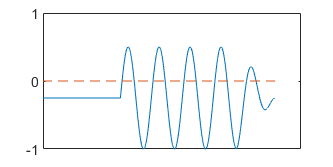• echoes—do not cause any problems, unless they manage to arrive before the test pulse;
• non-uniform group delay—it's a generic problem for any algorithm that uses a single frequency signal for latency detection. I guess, the transfer function needs to be measured before commencing latency testing;
• signal truncation—if the system "ramps up" the test signal, the algorithm will find the triggering threshold later, reporting an excessive latency.In fact, the last issue is a serious one. When doing manual testing, I always check the returned pulse visually, but the algorithm is "blind" to signal being ramped up. And ramping up can actually happen in mobile devices, where sophisticated processing is used for power saving and speaker protection purposes. Note that the algorithm can't use a "warm up" signal to put the system under measurement into a steady state because the warm up signal could be mistaken for the test pulse.

So, although a straightforward time domain approach has its strengths, it can be fooled, and a manual check of the results is required anyway.

I'm going to consider the methods used by other apps in following posts.